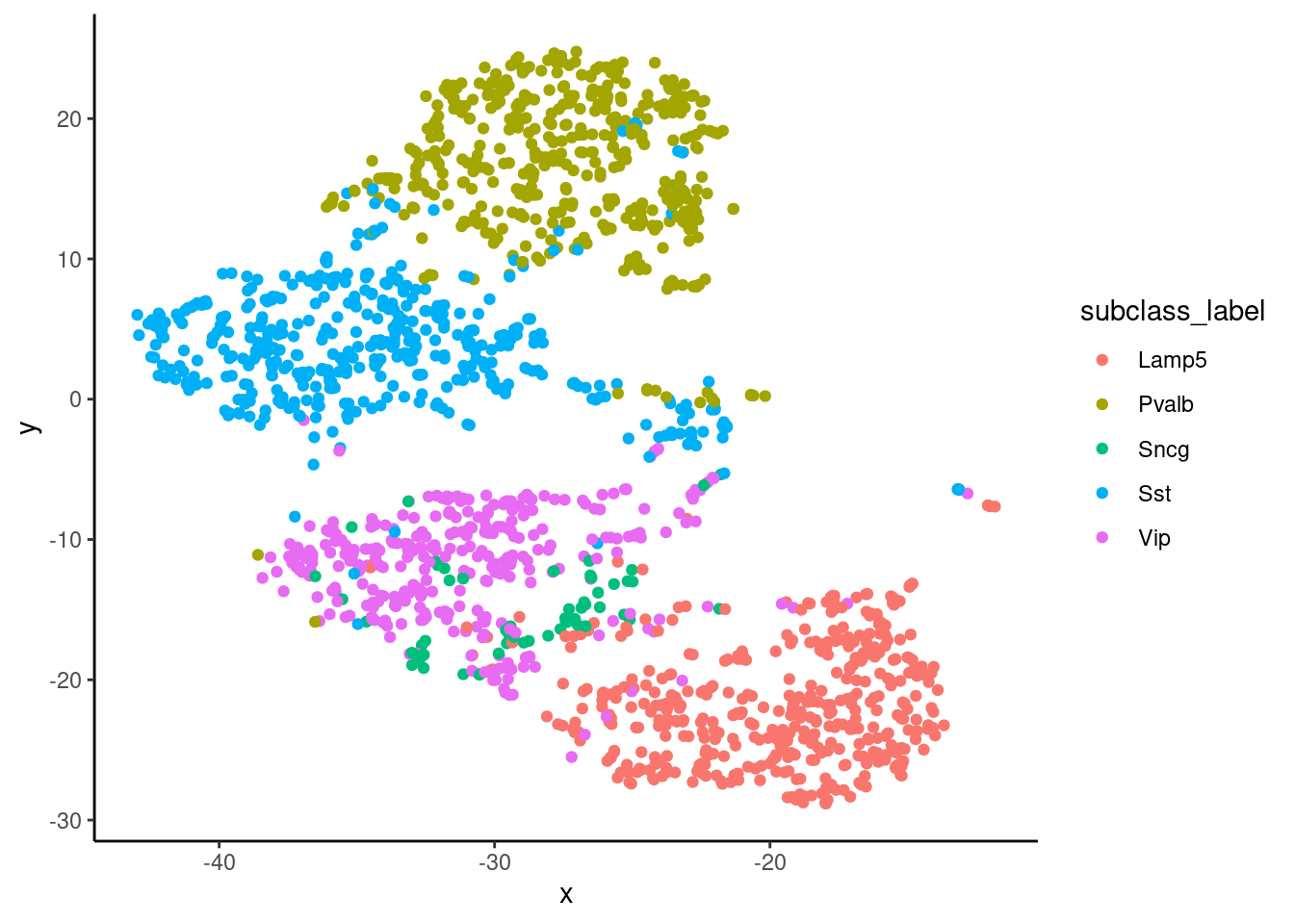# Installation

``````if (!requireNamespace("BiocManager", quietly = TRUE)) {
install.packages("BiocManager")
}
BiocManager::install("Dune")``````

We use a subset of the Allen Smart-Seq nuclei dataset. Run `?Dune::nuclei` for more details on pre-processing.

``````suppressPackageStartupMessages({
library(RColorBrewer)
library(dplyr)
library(ggplot2)
library(tidyr)
library(knitr)
library(purrr)
library(Dune)
})
data("nuclei", package = "Dune")
theme_set(theme_classic())``````

# Initial visualization

We have a dataset of \(1744\) cells, with the results from 3 clustering algorithms: Seurat3, Monocle3 and SC3. The Allen Institute also produce hand-picked cluster and subclass labels. Finally, we included the coordinates from a t-SNE representation, for visualization.

``````ggplot(nuclei, aes(x = x, y = y, col = subclass_label)) +
geom_point()``````We can also see how the three clustering algorithm partitioned the dataset initially:

``````walk(c("SC3", "Seurat", "Monocle"), function(clus_algo){
df <- nuclei
df\$clus_algo <- nuclei[, clus_algo]
p <- ggplot(df, aes(x = x, y = y, col = as.character(clus_algo))) +
geom_point(size = 1.5) +
# guides(color = FALSE) +
labs(title = clus_algo, col = "clusters") +
theme(legend.position = "bottom")
print(p)
})``````